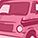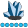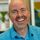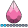Hey guys,

can we have a function named lead which does the opposite of lag.

data example;

input a b;

cards;

1 2

3 4

5 6

;

run;

data want;

set example;

c=lag(b);

run;

Ouput should look something like this

a b c d

1 2 . 4

3 4 2 6

5 6 4 .

Thanks@adityaa9z.  Beautiful dream, but I don't see it happening.

Consider this conditional use of lag, which gets within-store changes, where the stores are irregularly spaced in the data set.  Then think about what it would take to make the analogous within-store leads - SAS would have to read ahead an undetermined number of observations to get the lead value for same-store sales:

data have;

input store :\$1.   sales ;

select (store);

when ('A') prior_sales=lag(sales);

when ('B') prior_sales=lag(sales);

when ('C') prior_sales=lag(sales);

when ('D') prior_sales=lag(sales);

otherwise;

end;

datalines;

A 100

B 200

C 300

B 210

A 110

A 120

B 220

C 310

C 320

D 400

run;

This program creates 4 lag queues, one for each store.   Each queue is updated only when the corresponding store is current, which is why the prior_sales variable is always for the same store.  Combining them into a single

"when store in ('A','B','C','D') prior_sales=lag(sales);

would confound lags accross the 4 stores.

Other functions, that don't manage queues, would not need specific implementation for each store.  For example

when ('A','B','C','D') newvar=sqrt(sales)

works just as fine as having a when statement for each store.

Now doing the same for conditional leads (a lead "queue" I guess) would require, for each store, SAS to read ahead an undetermined number of records (after processing any where filters) until the same store is enountered: or if it's a LEAD2 function (2 periods) the same store would have to be enountered twice.   The whole remaining dataset would have to be read in those cases when a given store never reappears.  This is why we should always be mindful that the LAG function is a queue-management function, not just a look back like in excel.

However  ... these leads, as well as simpler leads, can be found via multiple SET statements, or MERGE statements.  You'd use the FIRSTOBS option, and possibly multiple WHERE conditions.  I'll be presenting these techniques in a Leads and Lags presentation at SGF2017 - also BASUG and NJSUG next month.

Bottom line.  As to a lead function - I don't see how it could be produced for regular DATA step programs.  For IML, yes, or other memory-resident objects (e.g. hash tables), but for the sequential access of most data steps, probably not.Super User

LAG also works in a data step while reading data. What would you expect the function to do when the data has not been read into SAS yet?Lapis Lazuli | Level 10

Could you just reverse the column and use a lag? In this example, one way would be sorting row a decending, then lagging b. It's another step, but it would work.@paulkaefer

Yes, the most common approach is to sort in descending order, do lags, and sort back to original order, effectively producing leads.

It's a big pain, and LOTS of disk input/output.  That's why I developed my presentation, which shows how to get leads without "resorting" to sort.Community Manager

SAS Technical Support gets this question a lot, and they prepared an article about a technique that you can use: LEAD and LAG at the same time.Rhodochrosite | Level 12

Hi,

I like to think of it this way...just as in life, the SAS data step is great at looking at "the past", but not so good at looking at "the future".  It's just an analogy, but it's how I look at it.

So, how do we derive lead?  Let's call this "fun with data structures".  (This also works with lag, but 1) SAS has a function for that, and 2) for a simple lag1, you can just assign the lag variable before calling the set statement; you don't need the lag function for that).

See below for various approaches, some better than others, esp. for large datasets.  Try to understand what each one is doing, and feel free to ping me with additional questions.

BTW, I have gotten this to work within groups as well (i.e. lead within groups), but since I didn't create suitable data to test that, I've left this out.  Essentially, use a BY statement or GROUP BY statement, with checks for first. and last. and judicious calls to call missing.

Hope this helps...

``````data have;
do key=65 to 90,97 to 122;
var=byte(key);
output;
end;
run;

* option 1: view + sql ;
data v / view=v;
n+1;
set have;
run;

proc sql;
create table want1 as
select a.key, a.var, b.var as lead_var, c.var as lag_var
from v a
full join v b
on a.n=b.n-1
full join v c
on a.n=c.n+1
where a.key is not missing
;
quit;

* option 2: interleave ;
data want2;
* set variable attributes (esp. var) ;
if 0 then set have;
lag_var=var;
merge have have (firstobs=2 keep=var rename=(var=lead_var));
run;

* option 3: multiple set statements (need to handle eof) ;
data want3;
* set variable attributes (esp. var) ;
if 0 then set have;
lag_var=var;
set have;
output;
if eof then do;
lag_var=var;
output;
end;
run;

* option 4: hash object ;
data want4;
* set variable attributes ;
if 0 then set v (rename=(n=_n var=_var));
if _n_=1 then do;
declare hash h (dataset:"v (rename=(n=_n var=_var))",hashexp:16);
h.defineKey("_n");
h.defineData("_var");
h.defineDone();
end;
call missing(of _all_);

* main record ;
set v;

* lag record ;
_n=n-1;
_rc=h.find();
if _rc=0 then lag_var=_var;

_n=n+1;
_rc=h.find();

drop _: n;
run;

* option 5: index ;
* this isn't good for large data, since the surrogate key (n) must be physically on the dataset ;* but it does illustrate random access via index + surrogate key ;
data have2 (index=(n));
set v;
run;

data want5;
* main record ;
set have2;

* save key var ;
_n=n;

* lag record ;
n=_n-1;
set have2 (keep=n var rename=(var=lag_var)) key=n;
if _iorc_ ne 0 then call missing(lag_var);

n=_n+1;
set have2 (keep=n var rename=(var=lead_var)) key=n;
if _iorc_ ne 0 then call missing(lead_var);

_error_=0;  * SAS treats a failed lookup as an error ;